## Understanding test results

In order to understand test results from standardized tests it is important to be familiar with a variety of terms and concepts that are fundamental to “measurement theory,” the academic study of measurement and assessment. Two major areas in measurement theory, reliability and validity, were discussed in the previous chapter; in this chapter we focus on concepts and terms associated with test scores.

## The basics

### Frequency distributions

A frequency distribution is a listing of the number of students who obtained each score on a test. If 31 students take a test, and the scores range from 11 to 30 then the frequency distribution might look like Table 1. We also show the same set of scores on a histogram or bar graph in Figure 1. The horizontal (or x-axis) represents the score on the test and the vertical axis (y-axis) represents the number or frequency of students. Plotting a frequency distribution helps us see what scores are typical and how much variability there are in the scores. We describe more precise ways of determining typical scores and variability next.

Table 1: Frequency distribution for 30 scores
Score on test Frequency Central tendency measures
17 1
18 1
19 0
20 3
21 2
22 6 Mode
23 3 Median
24 2 Mean
25 0
26 2
27 6 Mode
28 2
29 2
30 1
TOTAL 31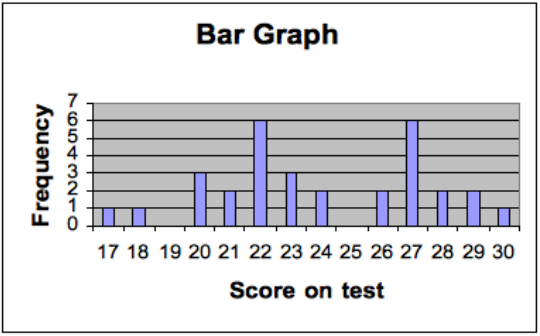Figure 1: Tests scores from Table 1 represented as a bar graph

## Central tendency and variability

There are three common ways of measuring central tendency or which score(s) are typical. The mean is calculated by adding up all the scores and dividing by the number of scores. In the example in Table 1, the mean is 24. The median is the “middle” score of the distribution—that is half of the scores are above the median and half are below. The median on the distribution is 23 because 15 scores are above 23 and 15 are below. The mode is the score that occurs most often. In Table 1 there are actually two modes: 22 and 27. Thus, this distribution is described as bimodal. Calculating the mean, median and mode are important as each provides different information for teachers. The median represents the score of the “middle” students, with half scoring above and below, but does not tell us about the scores on the test that occurred most often. The mean is important for some statistical calculations but is highly influenced by a few extreme scores (called outliers) but the median is not. To illustrate this, imagine a test out of 20 points taken by 10 students, and most do very well but one student does very poorly. The scores might be 4, 18, 18, 19, 19, 19, 19, 19, 20, 20. The mean is 17.5 (175/10) but if the lowest score (4) is eliminated the mean is now is 1.5 points higher at 19 (171/9). However, in this example the median remains at 19 whether the lowest score is included. When there are some extreme scores the median is often more useful for teachers in indicating the central tendency of the frequency distribution.

The measures of central tendency help us summarize scores that are representative, but they do not tell us anything about how variable or how spread out are the scores. Figure 2 illustrates sets of scores from two different schools on the same test for fourth graders. Note that the mean for each is 40 but in School A the scores are much less spread out. A simple way to summarize variability is the range, which is the lowest score subtracted from the lowest score. In School A with low variability the range is (45 − 35) = 10; in the school B the range is (55 − 22 = 33).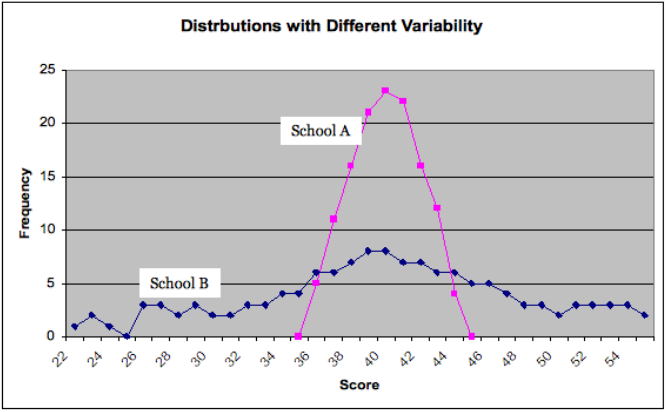Figure 2: Fourth grade math scores in two different schools with the same mean but different variability

However, the range is only based on two scores in the distribution, the highest and lowest scores, and so does not represent variability in all the scores. The standard deviation is based on how much, on average, all the scores deviate from the mean. In the example in Figure 2 the standard deviations are 7.73 for School A and 2.01 for School B. In Exhibit 1 below we demonstrate how to calculate the standard deviation.

### Exhibit 1: Calculating a standard deviation

The scores from 11 students on a quiz are 4, 7, 6, 3, 10, 7, 3, 7, 5, 5, and 9. Complete the following steps:

1. Order scores.
2. Calculate the mean score.
3. Calculate the deviations from the mean.
4. Square the deviations from the mean.
5. Calculate the mean of the squared deviations from the mean (i.e. sum the squared deviations from the mean then divide by the number of scores). This number is called the variance.
6. Take the square root and you have calculated the standard deviation.

#### Completing the Steps

Score (Step 1: Order) Deviation from the mean Squared deviation from the mean
3 −3 9
3 −3 9
4 −2 4
5 −1 1
5 −1 1
6 0 0
7 1 1
7 1 1
7 1 1
9 3 9
10 4 4
Total: 66  52
##### Step 2: Calculate Mean

$\displaystyle\text{mean}=\frac{66}{11}=6\\$

##### Step 3: Calculate Deviations

$\displaystyle\text{mean}=\frac{40}{11}=4.73\\$

##### Step 4–5: Complete the Calculations

$\displaystyle\text{Standard deviation}=\frac{\sqrt{\sum\left(\text{score}-\text{mean}\right)^2}}{N}\\$

N = Number of scores

##### Step 6: Find the Standard Deviation

$\displaystyle\text{Standard deviation}=\sqrt{4.73}=2.17\\$

## The normal distribution

Knowing the standard deviation is particularly important when the distribution of the scores falls on a normal distribution. When a standardized test is administered to a very large number of students the distribution of scores is typically similar, with many students scoring close to the mean, and fewer scoring much higher or lower than the mean. When the distribution of scores looks like the bell shape shown in Figure 3 it is called a normal distribution. In the diagram we did not draw in the scores of individual students as we did in Figure 2, because distributions typically only fall on a normal curve when there are a large number of students; too many to show individually. A normal distribution is symmetric, and the mean, median and mode are all the same.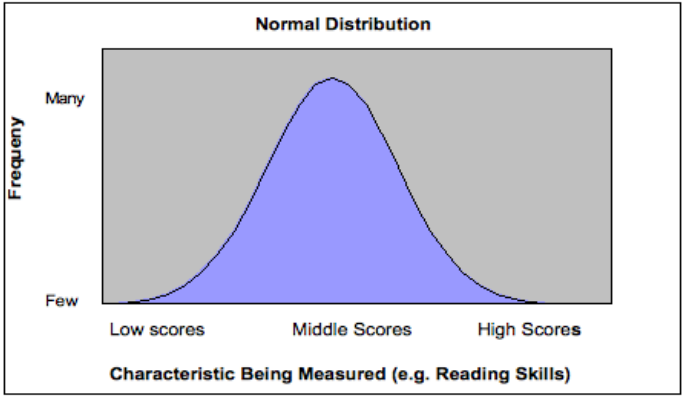Figure 3: Bell shaped curve of normal distribution

Normal curve distributions are very important in education and psychology because of the relationship between the mean, standard deviation, and percentiles. In all normal distributions 34 percent of the scores fall between the mean and one standard deviation of the mean. Intelligence tests often are constructed to have a mean of 100 and standard deviation of 15 and we illustrate that in Figure 4.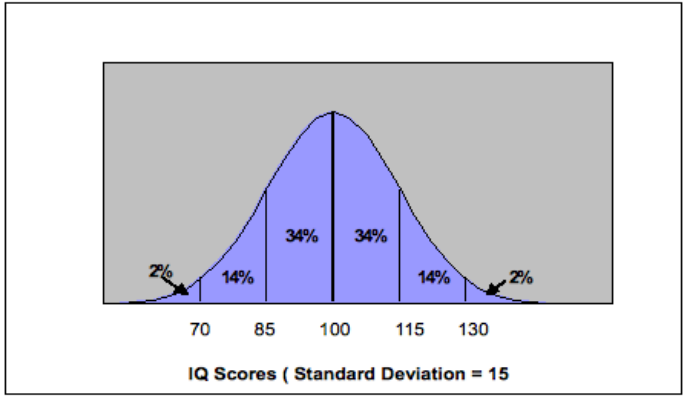Figure 4: Normal distribution for an IQ test with mean 100 and standard deviation 15

In Figure 4, 34 percent of the scores are between 100 and 115 and as well, 34 percent of the scores lie between 85 and 100. This means that 68 percent of the scores are between -1 and +1 standard deviations of the mean (i.e. 85 and 115). Note than only 14 percent of the scores are between +1 and +2 standard deviations of the mean and only 2 percent fall above +2 standard deviations of the mean.

In a normal distribution a student who scores the mean value is always in the fiftieth percentile because the mean and median are the same. A score of +1 standard deviation above the mean (e.g. 115 in Figure 4) is the 84 percent tile (50 percent and 34 percent of the scores were below 115). In Exhibit 10 we represent the percentile equivalents to the normal curve and we also show standard scores.

## Kinds of test scores

A standard score expresses performance on a test in terms of standard deviation units above of below the mean (Linn & Miller, 2005). There are a variety of standard scores, including z-scores, T-scores, and stanines.

One type of standard score is a z-score, in which the mean is 0 and the standard deviation is 1. This means that a z-score tells us directly how many standard deviations the score is above or below the mean. For example, if a student receives a z score of 2 her score is two standard deviations above the mean or the eighty-fourth percentile. A student receiving a z score of -1.5 scored one and one half deviations below the mean. Any score from a normal distribution can be converted to a z score if the mean and standard deviation is known. The formula is:

$\displaystyle\text{z-score}=\frac{\text{score }-\text{ mean score}}{\text{standard deviation}}\\$

So, if the score is 130 and the mean is 100 and the standard deviation is 15 then the calculation is:

$\displaystyle\text{Z}=\frac{130-100}{15}=2\\$

If you look at Figure 4 you can see that this is correct—a score of 130 is 2 standard deviations above the mean and so the z score is 2.

A T-score has a mean of 50 and a standard deviation of 10. This means that a T-score of 70 is two standard deviations above the mean and so is equivalent to a z-score of 2.

Stanines (pronounced “staynines”) are often used for reporting students’ scores and are based on a standard nine point scale and with a mean of 5 and a standard deviation of 2. They are only reported as whole numbers and Figure 11-10 shows their relation to the normal curve.

Testing companies calculate grade equivalents by giving one test to several grade levels. For example a test designed for fourth graders would also be given to third and fifth graders. The raw scores are plotted and a trend line is established and this is used to establish the grade equivalents. Note that in Figure 5 the trend line extends beyond the grades levels actually tested so a grade equivalent above 5.0 or below 3.0 is based solely on the estimated trend lines.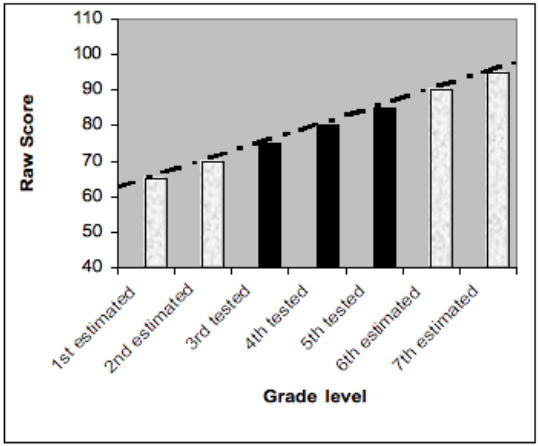Figure 5: Using trend lines to estimate grade equivalent scores.

Grade equivalent scores also assume that the subject matter that is being tested is emphasized at each grade level to the same amount and that mastery of the content accumulates at a mostly constant rate (Popham, 2005). Many testing experts warn that grade equivalent scores should be interpreted with considerable skepticism and that parents often have serious misconceptions about grade equivalent scores. Parents of high achieving students may have an inflated sense of what their child’s levels of achievement.

## References

Linn, R. L., & Miller, M. D. (2005). Measurement and Assessment in Teaching 9th ed. Upper Saddle River, NJ: Pearson.

Popham, W. J. (2005). Classroom Assessment: What teachers need to know. Boston:, MA: Pearson.